ZSAIm 我也不知道是什么攻城狮

# 爱奇艺视频cmd5x解析算法的移植分析和实现<Nodejs>（2019-08）。

## 说明

1. 这不是完整的分析视频解析的过程，如果需要了解整一个大概过程可以参考2018版的爱奇艺视频解析分析过程。下面文章将直接以`cmd5x`代码为起点进行分析。

2. 这是`2019-08`版本的cmd5x算法的分析处理思路。

3. 由于算法太臃肿，所以下面的分析讲通过贴出`伪代码`来进行分析，如果需要完整的算法可以点这里
4. 这文章的分析不是对算法的简化分析，而是为了在非浏览器端nodejs实现cmd5x算法，也就是从浏览器端移植到Nodejs。

## 大概

• 进行了方法名的混淆。
• 引入了浏览器端天生具有的而Nodejs这些运行时天生所不具有的document和window。
• 进行了非浏览器端JS运行时的识别（如Nodejs中的特有指令process和require的判断）。

## 分析过程

### 首先

``````function(module, exports, __webpack_require__) {
var _qda = [...];	// 混淆方法名
!function(e, t) {
!function(t) {
for (; --t; )
e.push(e.shift())
}(++t)
}(_qda, 115);		// 混淆偏移
var qdb = function(e, t){
// 包含document, window的算法执行代码。
};
function _qd_az() {
// 包含document, window的初始化代码。
}
_qd_az();
}
``````

``````var r = e.url.replace(new RegExp("^(http|https)://" + t,"ig"), "");
d && (r = r.replace("/3ea/420a8433732a6c99d1eae98fea69e55d", "")),
n = s.a.cmd5x(r)
``````

### 方案

1. 暴力破解：
• 既然cmd5x是一个加了盐的md5算法，那么应该是可以使用遍历所加的盐来暴力破解，但是这计算量确实是非常不友好，这从来都是一个万不得已的解决方法。
2. 暴力调试。
• 所谓的暴力调试就是通过比较输出循环结果来找出并以此来解决不匹配的点，以便于修正不匹配的问题，事实上这跟通过print来调试程序bug的本质是一致的，暴力调试是一个相对一个比较通用的解决方法。

### 关于调试

#### 如何使用chromium的开发者工具对本地导入的代码进行断点调试

•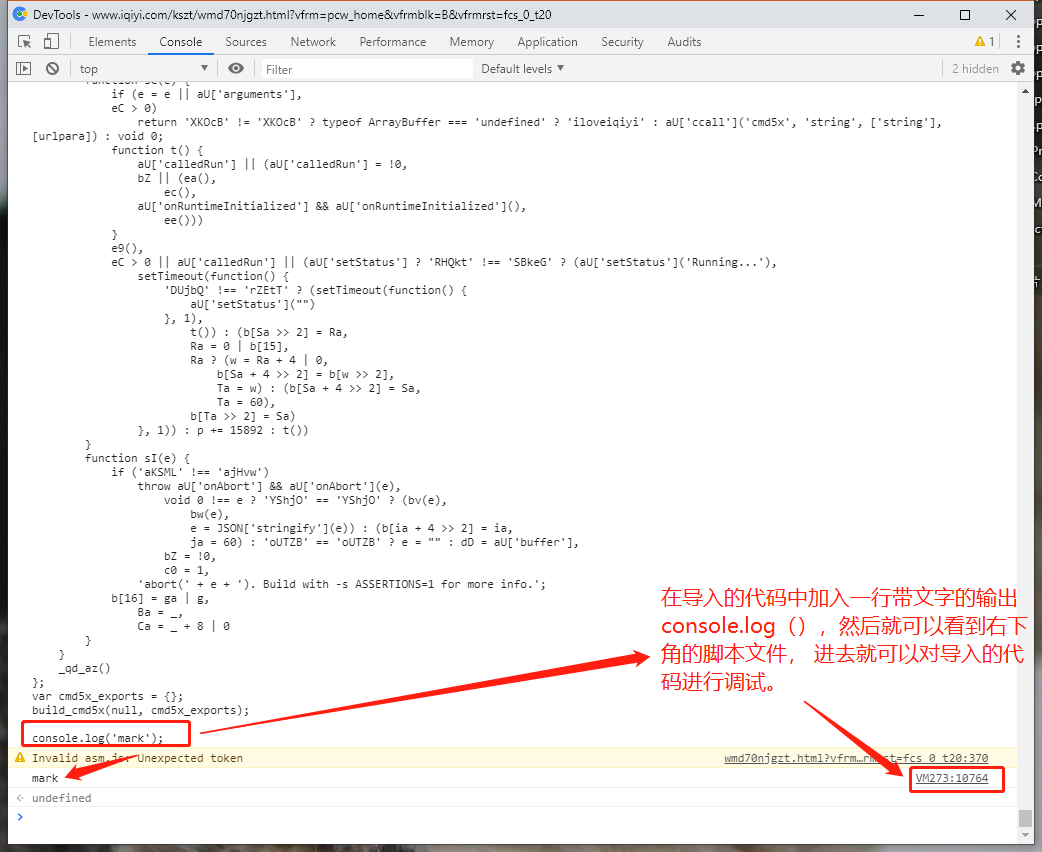•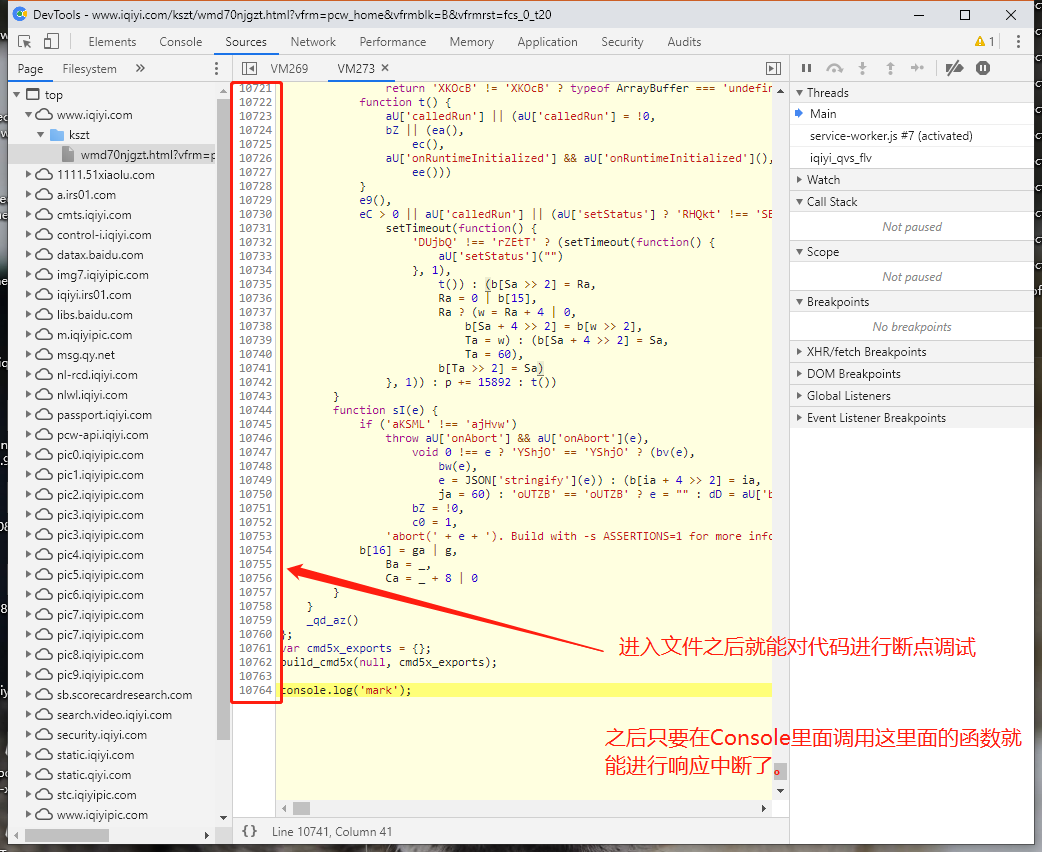### 第一次测试

• 既然我们已经找到了cmd5x实现的算法，我们在进行第一次调试是否能够正常使用，

• 我们的目标是在Nodejs上实现cmd5x，所以我们对找到的cmd5x代码放到Nodejs里面运行一下看下代码是否侥幸的运行正确。

• 为了在nodejs中运行，建立如下代码。（伪代码）

• ``````var build_cmd5x = function(module, exports, __webpack_require__) {
var _qda = [...];	// 混淆方法名
!function(e, t) {
!function(t) {
for (; --t; )
e.push(e.shift())
}(++t)
}(_qda, 115);		// 混淆偏移
var qdb = function(e, t){
// 包含document, window的算法执行代码。
};
function _qd_az() {
// 包含document, window的初始化代码。
}
_qd_az();
}
var cmd5x_exports = {};
build_cmd5x(null, cmd5x_exports);
``````
• 放进nodejs执行发生如下错误。

• ``````            Ca = 60) : (b2 ? b5 = self[_qdb("0x29")][_qdb("0x2a")] : document[_q
db("0x2b")] && (b5 = document[_qdb("0x2b")][_qdb("0x2c")]),
^

ReferenceError: document is not defined
at startup (internal/bootstrap/node.js:283:19)
``````

### 模拟环境测试(可选)

• 到目前为止我们发现并不能简单的扣代码运行，其中的`document, window`是浏览器端先天具有而非浏览器端运行时如nodejs不先天具有的对象。 这个问题第一反应是先借助nodejs的第三方库构建`window 和 document`对象（这里我们使用库`jsdom`），之后当实现了目的后，如果有进一步的需求，我们可以再将库jsdom的依赖去掉（实际上算法并不需要依赖`document 和 window`，或许可能仅仅依赖其中一些数据。一步一步排错修改，这样就能避免过多的变量造成调试的困难）。
• 要注意的是，jsdom所构造得到的window和document并不能完全构造在浏览器端运行的window和document，所以这之后将是要考虑的一个问题。

• `build_cmd5x`之前加入如下代码来构建`window 和 document`

• ``````const jsdom = require('jsdom');
const { JSDOM } = jsdom;

const dom = new JSDOM(``, {
url: "http://www.iqiyi.com/",
referrer: "http://www.iqiyi.com/",
contentType: "text/html",
includeNodeLocations: true,
storageQuota: 1000000
});

global.window = dom.window;
global.document = window.document;

global.self = window;
document.domain = "iqiyi.com";

...

``````
• “安全”通过`build_cmd5x`，之后我们继续测试执行`cmd5x`来看下输出值是否是所需要的。

• 为了检验cmd5x的正确性，我们在浏览器端的运行结果`cmd5x("/dash?tvid=2185399200&bid=300&vid=52ec66e4dc5263184c5b1b378ee6eb81&src=01010031010000000000&vt=0&rs=1&uid=&ori=pcw&ps=0&k_uid=be720e39e62fd602f95e957dc401891e&pt=0&d=0&s=&lid=&cf=&ct=&authKey=7ebb8c20728f94c13e74f3aa555f48e6&k_tag=1&ost=0&ppt=0&dfp=a0aadc0b7de24f4e3b97fe7d20230fbce8b3b1c4d0d9aa9904353e363ea4ece6ea&locale=zh_cn&prio=%7B%22ff%22%3A%22f4v%22%2C%22code%22%3A2%7D&pck=&k_err_retries=0&up=&qd_v=2&tm=1566399701976&qdy=a&qds=0&k_ft1=141287244169220&k_ft4=8196&k_ft5=1&bop=%7B%22version%22%3A%2210.0%22%2C%22dfp%22%3A%22a0aadc0b7de24f4e3b97fe7d20230fbce8b3b1c4d0d9aa9904353e363ea4ece6ea%22%7D&ut=0") = 8f2b8b04a29e5e8d0f10b8e7a7bc7668`来进行首次检验运行的正确性。

• ``````r = "/dash?tvid=2185399200&bid=300&vid=52ec66e4dc5263184c5b1b378ee6eb81&src=01010031010000000000&vt=0&rs=1&uid=&ori=pcw&ps=0&k_uid=be720e39e62fd602f95e957dc401891e&pt=0&d=0&s=&lid=&cf=&ct=&authKey=7ebb8c20728f94c13e74f3aa555f48e6&k_tag=1&ost=0&ppt=0&dfp=a0aadc0b7de24f4e3b97fe7d20230fbce8b3b1c4d0d9aa9904353e363ea4ece6ea&locale=zh_cn&prio=%7B%22ff%22%3A%22f4v%22%2C%22code%22%3A2%7D&pck=&k_err_retries=0&up=&qd_v=2&tm=1566399701976&qdy=a&qds=0&k_ft1=141287244169220&k_ft4=8196&k_ft5=1&bop=%7B%22version%22%3A%2210.0%22%2C%22dfp%22%3A%22a0aadc0b7de24f4e3b97fe7d20230fbce8b3b1c4d0d9aa9904353e363ea4ece6ea%22%7D&ut=0";
console.log(cmd5x_exports.cmd5x(r));
``````
• 上面的代码运行得到`f48f5790c89d585630a083e3ce052442`。很显然这并不是算法得到的结果。
• 为什么一样的代码得到不一样的结果，而不是报错。这或许说明了存在着一个或多个“开关”——能够使得在浏览器端与非浏览器端中进行切换算法，使得输出的结果不一样。

• 到目前为止我们不能仅通过宏观的处理实现，所以后面需要分析到具体代码了。但是分析代码对于小型的代码可以应付得来，但是对于几万行的代码来说绝不是小事。

### 初看代码结构

• ``````function(module, exports, __webpack_require__) {
var _qda = [...];	// 混淆方法名
!function(e, t) {
!function(t) {
for (; --t; )
e.push(e.shift())
}(++t)
}(_qda, 115);		// 混淆偏移
var qdb = function(e, t){
// 包含document, window的算法执行代码。
};
function _qd_az() {
// 包含document, window的初始化代码。
}
_qd_az();
}
``````
• 第一次大概调试走一遍算法。然后会发现其中存在着很多的`_qdb(0x?)`，看到这里我们调试走进去出来之后得到的是一个字符串`length`或者`name`这些常见的对象的属性，可以猜测这是一种混淆的方法。
• 我们走进去`_qdb()`函数发现了它依赖于`_qda`，所以有理由的怀疑这是`_qdb()`是用来解析`_qda`变量的元素的。可以反复的给`_qdb`传参来调用如 `_qdb('0x0')`来测试函数`_qdb`的输出结果是否受调用次数的影响（当然这也可以通过粗略看`_qdb`代码来知道这一点）。
• 结果就是`_qdb()`调用结果不受调用次数影响，所以我们可以将所有的混淆替换一下文本来进行简单的反混淆。

#### 反混淆

• 有很多方法可以实现文本的替换，这里我使用`python`实现一下批量替换。

• 首先我们先在脚本中输出替换文本和被替换文本。注意到变量`_qda`的定义后面有一个自执行函数，是用来左移数组变量`_qda`的，所以我们需要在这之后运行。

• ``````// 其中 _qda.length = 1312
var kv = [];
for(var i=0; i<1312; i++){
kv.push(i.toString(16) + '|' + _qdb('0x' + i.toString(16)));
}
console.log(kv.join('\n'));
// 之后将输出写出文件
``````
• 保存输出结果。

• 使用如下代码得到的替换后的代码。

• ``````# 假设源文本读入变量	origin_txt,
# 替换文本读入变量	replace_txt
replace_list = []
for i in replace_txt.strip().split('\n'):
replace_list.append(i.split('|'))

retval = origin_txt
for i in replace_list:
retval = retval.replace('_qdb(0x%s)' % i, i.__repr__())

# 写出 retval 到文件，替换新的build_cmd5x
``````

### 测试反混淆结果

• 为了保险起见，对于反混淆的结果后我们需要测试一下是否对算法结果有影响。
• 在浏览器端的环境进行运行一下来看下是否正确。
• 在浏览器任意打开一个爱奇艺视频，进入开发者工具F12（这里使用百分浏览器），进入`Console`，将代码放进去运行一下。然后看下结果。
•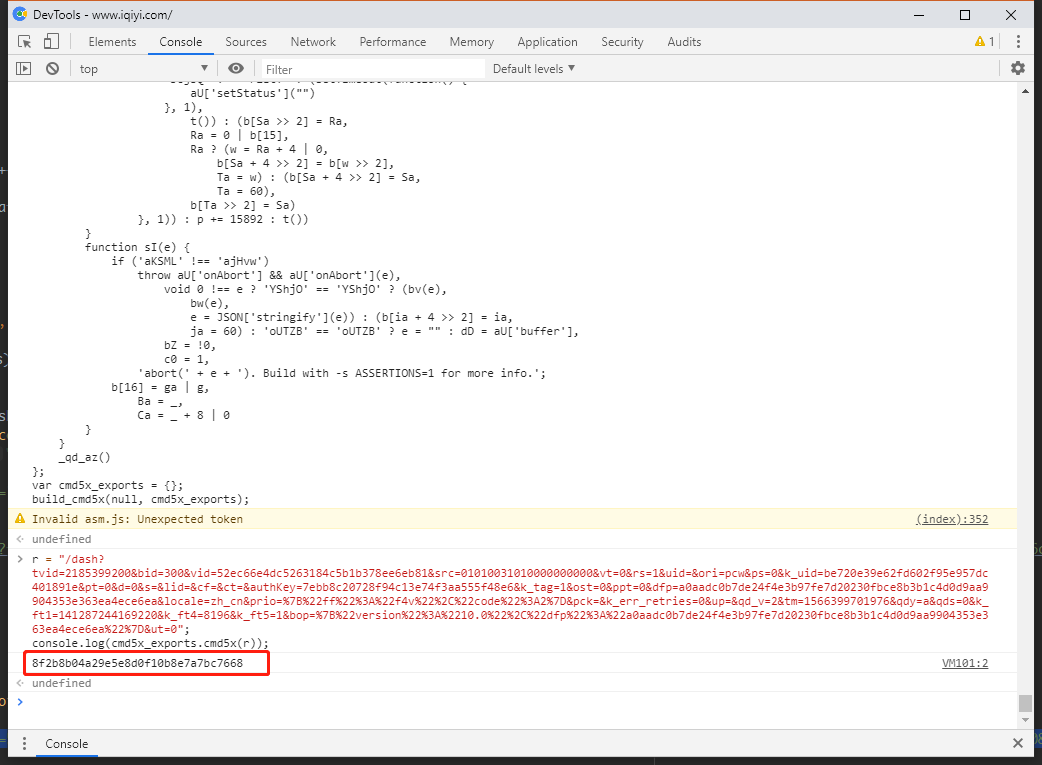• 除此之外还可以在其他网站运行这样的代码。下面我们在www.baidu.com和www.bilibili.com下运行了这段代码。
•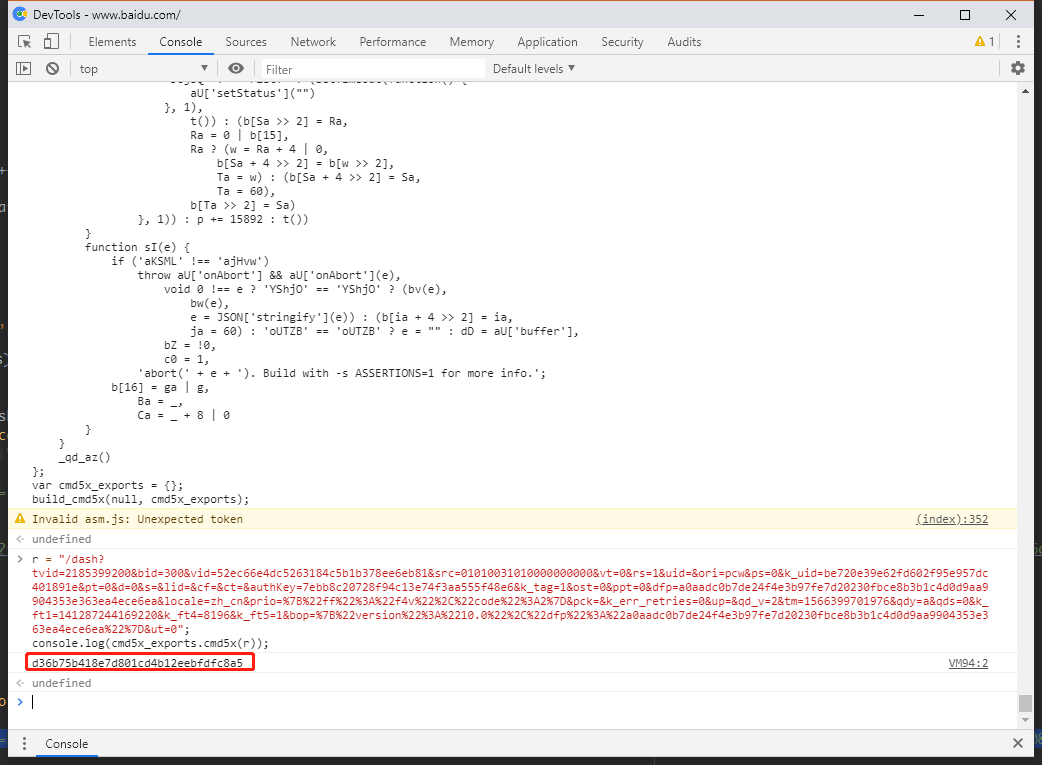,
•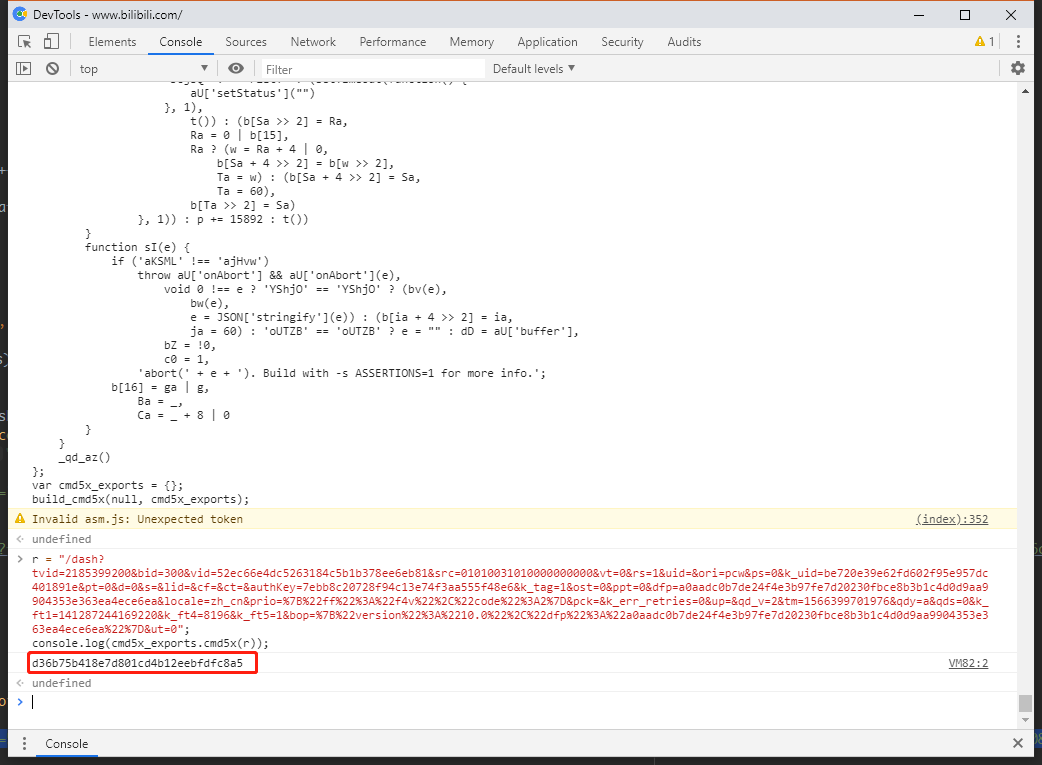#### 为什么这么做？

• `iqiyi`视频页处运行这段代码用意：
• 测试反混淆代码是否正确，以便于后面工作的执行。
• 测试是否在其他地方进行算法的额外初始化工作。（其他地方进行额外初始化工作的意思是代码中可能存在export到window或其他全局变量，然后在其他JS脚本进行调用初始化的函数。）
• 在其他网站页如`bilibili``baidu`处运行这段代码用意：
• 测试算法的运行结果是否与document和window里面的一些数据有关系。

#### 这样的结果说明了什么？

• 反混淆代码是正确的。
• 没有在其他地方的额外初始化工作。

• 算法的运行确实与document和window的数据有关系。
• 可以推测的是这种关系是一种数据判断关系，算法本身并不需要这些数据。这我们可以通过在两个不同的网站里面得到了一样的结果知道这些。

### 暴力调试

#### 怎么调试呢？

• 通过调试`n = s.a.cmd5x(r)`我们可以知道的是其函数所在就是：

• ``````aF = function(e) {
if ('mwlCr' !== 'UdOHx')
return typeof ArrayBuffer === 'undefined' ? 'iloveiqiyi' : aU['ccall']('cmd5x', 'string', ['string'], [e]);
b[na >> 2] = ma,
ma = 0 | b,
ma ? (w = ma + 4 | 0,
b[na + 4 >> 2] = b[w >> 2],
oa = w) : (b[na + 4 >> 2] = na,
oa = 60),
b[oa >> 2] = na
}
``````
• 必然的是会执行`return typeof ArrayBuffer === 'undefined' ? 'iloveiqiyi' : aU['ccall']('cmd5x', 'string', ['string'], [e]);`

• ``````function c7(e, t, n, r, i) {
var o = {};

...

return f = d,
d = t === 'string' ? cM(f) : t === 'boolean' ? Boolean(f) : f,
0 !== u && sg(u),
d
}
``````
• `build_cmd5x`的时候会执行初始化工作`_qd_az()`，我们进去走一篇可以发现的是算法使用了`ArrayBuffer`。如下代码：

• ``````function dM() {
'gNDMI' != 'gNDMI' ? document['title'] = title : (aU['HEAP8'] = dE = new Int8Array(dD),
aU['HEAP16'] = dG = new Int16Array(dD),
aU['HEAP32'] = dI = new Int32Array(dD),
aU['HEAPU8'] = dF = new Uint8Array(dD),
aU['HEAPU16'] = dH = new Uint16Array(dD),
aU['HEAPU32'] = dJ = new Uint32Array(dD),
aU['HEAPF32'] = dK = new Float32Array(dD),
aU['HEAPF64'] = dL = new Float64Array(dD))
}
``````
• 我们可以看到`Buffer``dD`。所以`dE, dG, dI, dF, dH, dJ, dK, dL`是绑在`dD`的。所以我们可以选择其中一个进行数据对比， 如`dJ`
• 首先如果是几千或者几万个数据当然你可以将每一个数据输出进行比较。但是这么是几十万几百万，电脑可能就吃不消，所以这就不适合将所有数据输出来比较。

• 由于未初始化数据都是`0`，所以我们可以仅仅输出非`0`的数据，这样就能大大减少比较的数据量。

• 如下函数用于输出待比较的数据。

• ``````function test_arraybuffer(arraydata){
for(var i=0; i<arraydata.length; i++){
if(data != 0){
print_data.push([i, data].join(' '));
}
}
}

...

console.log(print_data.join('\n'));
``````
• 我们再回去看函数`c7()`， 比较一下在浏览器端和nodejs输出的数据是否匹配。如果不匹配就是说明前面存在使他们不匹配的数据，这才是我们关注的对象。也就是说我们需要逐步缩小不匹配问题的所在地点，然后重点分析。

• ``````function c7(e, t, n, r, i) {
var o = {};
o['string'] = function(e) {
if ('dDZQD' !== 'HIUzl') {
var t = 0;
if (null != e && 0 !== e) {
var n = 1 + (e.length << 2);
d4(e, t = sf(n), n)
}
return t
}
b[ib + 4 >> 2] = ib,
jb = 60
}
,
o['array'] = function(e) {
var t = sf(e.length);
return dh(e, t),
t
}
;
/// 1号
var a = c4(e)
, s = []
, u = 0;
// 2号
if (r)
for (var c = 0; c < r['length']; c++) {
var l = o[n[c]];
l ? (0 === u && (u = sh()),
s[c] = l(r[c])) : s[c] = r[c]
}
// 3号
var f, d = a['apply'](null, s);
// 4号
return f = d,
d = t === 'string' ? cM(f) : t === 'boolean' ? Boolean(f) : f,
0 !== u && sg(u),
d
}
``````
• 我们注意上面代码的`1~4号`，我们在这些地方可以分别加上`test_arraybuffer(dJ);`，并将在`浏览器端和nodejs端`的输出数据分别进行比较。如果出现不一致的问题就说明了之间有不匹配的点，而那些所谓的浏览器端和nodejs的`识别开关`就存在于其中。
• 下面给出在一号位在浏览器端和Nodejs端的结果的比较。

•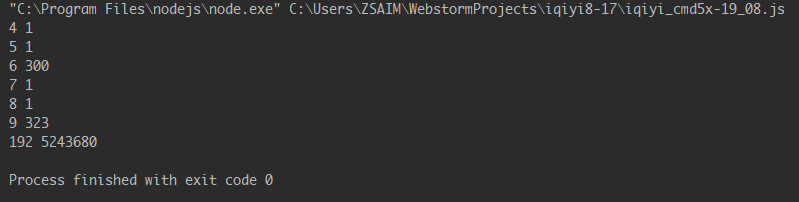,
•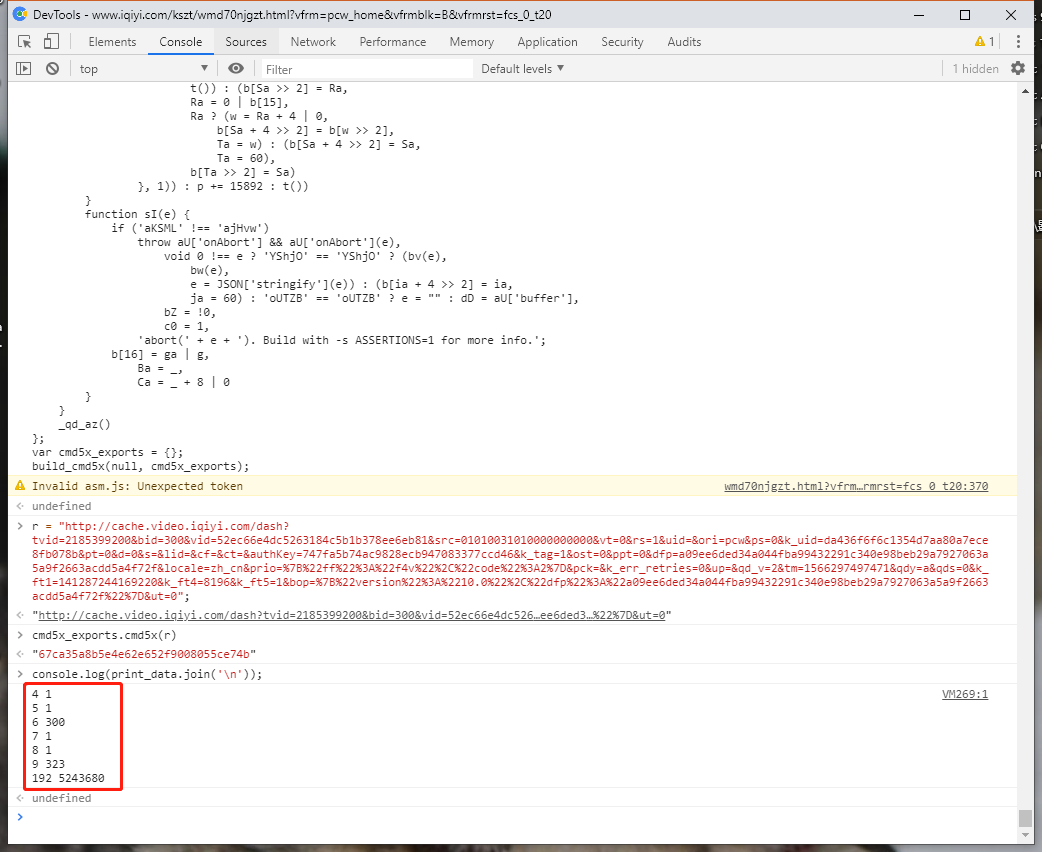,
•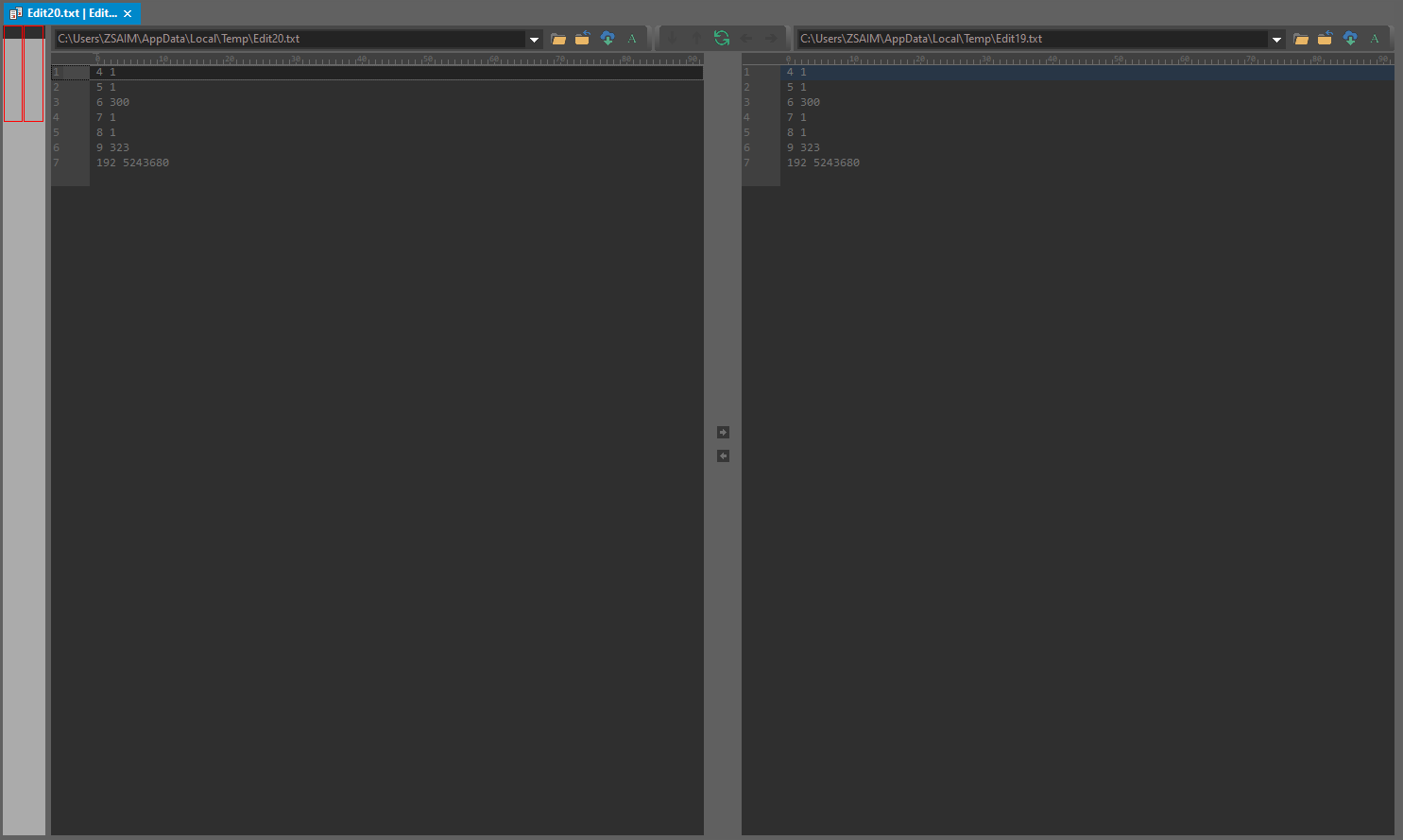• 然后我就不一一列出来比较结果，这里直接说结果就是`识别开关`就存在于`var f, d = a['apply'](null, s);`（因为在3号位数据一致，4号位数据不一致）。

• 我们进去`var f, d = a['apply'](null, s);`

• ``````function hn(e) {
...
// ArrayBuffer 数据一致。
e: for (; ; )
if ('TpEqY' === 'guQVq')
b = Pe + 4 | 0,
gL[Ae + 4 >> 2] = gL[b >> 2],
qe = b;
else {
switch ((255 & x) << 24 >> 24) {
...
}
Q = ht,
z = pt,
G = _t,
H = gt,
W = yt,
V = bt,
B = vt,
F = mt,
j = kt,
N = wt,
U = St,
M = xt,
C = Tt,
R = Lt,
D = Et,
I = Pt,
O = At,
q = qt,
A = Ot,
P = It,
E = Dt,
L = Rt,
T = Ct,
x = Mt,
S = Ut
}

...
}
``````
• 同样的方法，我们先比较`ArrayBuffer`的数据。之后我们会发现一直到`e: for(;;)`数据还是一致。

• 这又遇到了麻烦了。因为下面就是算法的最后一步，而这一步是一个无限循环，代码又是一长串，我们不希望在每一种case都进行比较一下ArrayBuffer，第一这既繁琐，第二这又无法得知是在第几次循环后的case。

• 所以之后我们应该转向考察其他的变量，找那些for循环里面用到的东西，如上面这些变量，同时计数一下，用于找出循环次数是否一样。

• ``````function hn(e) {
...
// 计数变量 counter;
var counter = 0;
e: for (; ; )
if ('TpEqY' === 'guQVq')
b = Pe + 4 | 0,
gL[Ae + 4 >> 2] = gL[b >> 2],
qe = b;
else {
// 如下代码将用来输出比较数据
counter++;
print_data.push([counter,lt,ct,ut,b,at,ot,it,rt,nt,tt,\$e,Je,Ze,Xe,Ke,Ye,Qe,ze,Ge,He,We,Ve,Be,Fe,je,Ne,Ue,Me,Ce,Re,De,Ie,Oe,qe,Ae,Pe,Ee,Le,Te,xe,Se,we,ke,me,ve,be,ye,ge,_e,pe,he,de,fe,le,ce,ue,se,ae,oe,ie,re,ne,te,ee,\$,J,Z,X,K,Y,Q,z,G,H,W,V,B,F,j,N,U,M,C,R,D,I,O,q,A,P,E,L,T,x].join(' '));

// 第二部可以如下调试，for循环里面所经过的路径
print_data.push([counter, (255 & x) << 24 >> 24].join(' '))

switch ((255 & x) << 24 >> 24) {
...
}
Q = ht,
z = pt,
G = _t,
H = gt,
W = yt,
V = bt,
B = vt,
F = mt,
j = kt,
N = wt,
U = St,
M = xt,
C = Tt,
R = Lt,
D = Et,
I = Pt,
O = At,
q = qt,
A = Ot,
P = It,
E = Dt,
L = Rt,
T = Ct,
x = Mt,
S = Ut
}

...
}
``````
• 当然也可以从for循环里面的的代码发现，里面大都是数学运算，只有少少的几条函数调用，就可以发现下面这几条，或许就是非浏览器端识别的关键所在。

• ``````// 第一条
w = 0 | s1[1 & gL[16 + ((0 != (0 | gL) & 1) + (0 == (0 | gL) & 1) << 2) >> 2]]();
// 第二条
m = 0 | rb(0 | gL[(w = i + (z << 3) | 0) >> 2], 0 | gL[w + 4 >> 2], 0 | r6(0 | k, ((0 | k) < 0) << 31 >> 31 | 0, 1), 0 | h0());
// 第三条
yt = v = 6 + ((w = (0 | z) % 4 | 0) << 2) + ((0 | gX(w + -1 | 0, w)) / 2 | 0) | 0;
// 第四条
s3[1 & gL[16 + ((0 != (0 | gL) & 1) + ((0 == (0 | y)) << 31 >> 31) + ((0 != (0 | y) & 1) << 1) << 2) >> 2]](S);
// 第五条
s2[1 & gL[16 + (((0 == (0 | gL) & 1) << 1 | 4) << 2) >> 2]](33);
// 第六条
k = 0 | rb(0 | gL[(y = i + (z << 3) | 0) >> 2], 0 | gL[y + 4 >> 2], 0 | r6(0 | v, ((0 | v) < 0) << 31 >> 31 | 0, 1), 0 | h0());
....
``````
• 函数`h0()`, `s1[...]()`, `s2[...]()`, `s3[...]()`, `gX()`, `r6()`…可以逐一去调试进去看一下。
•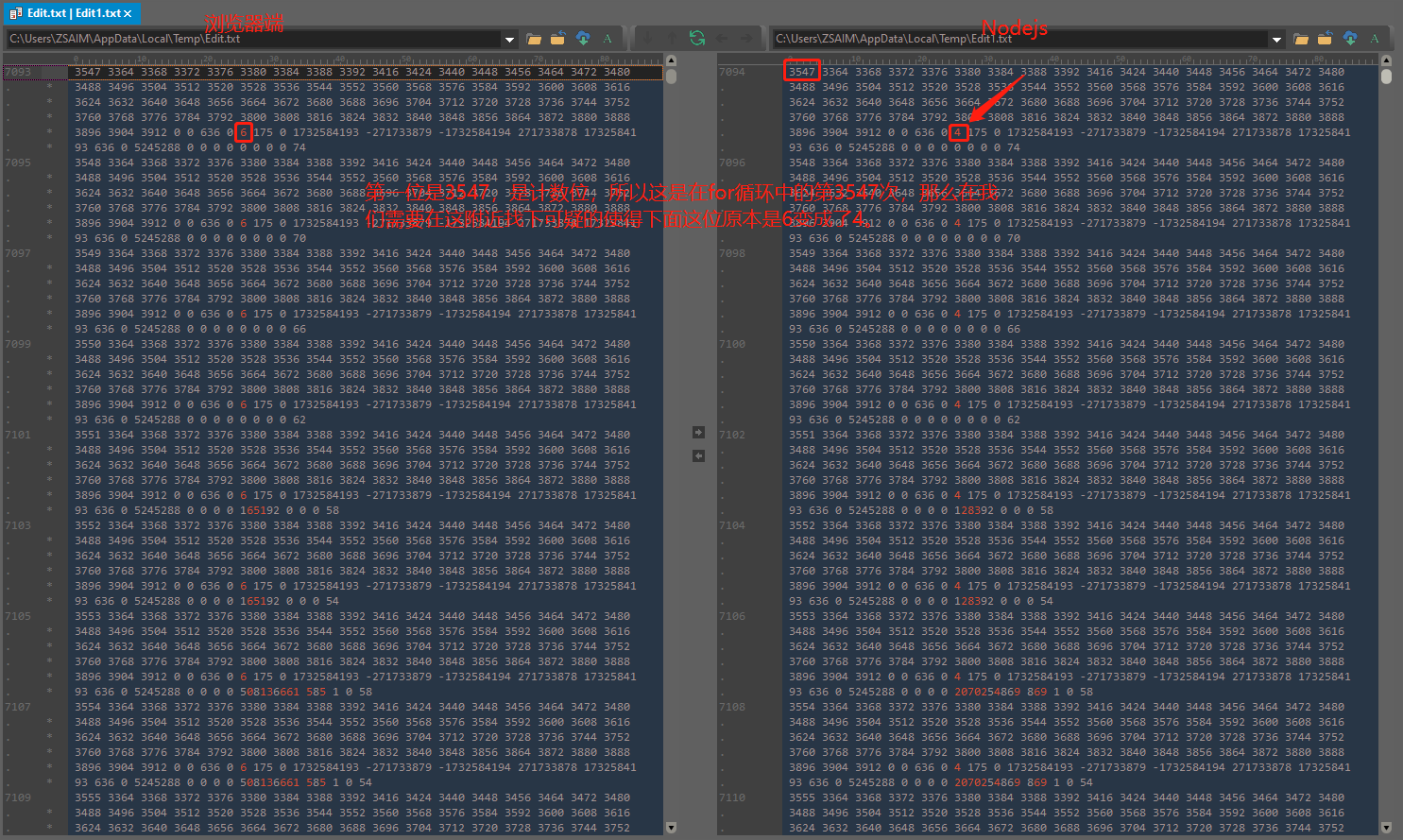•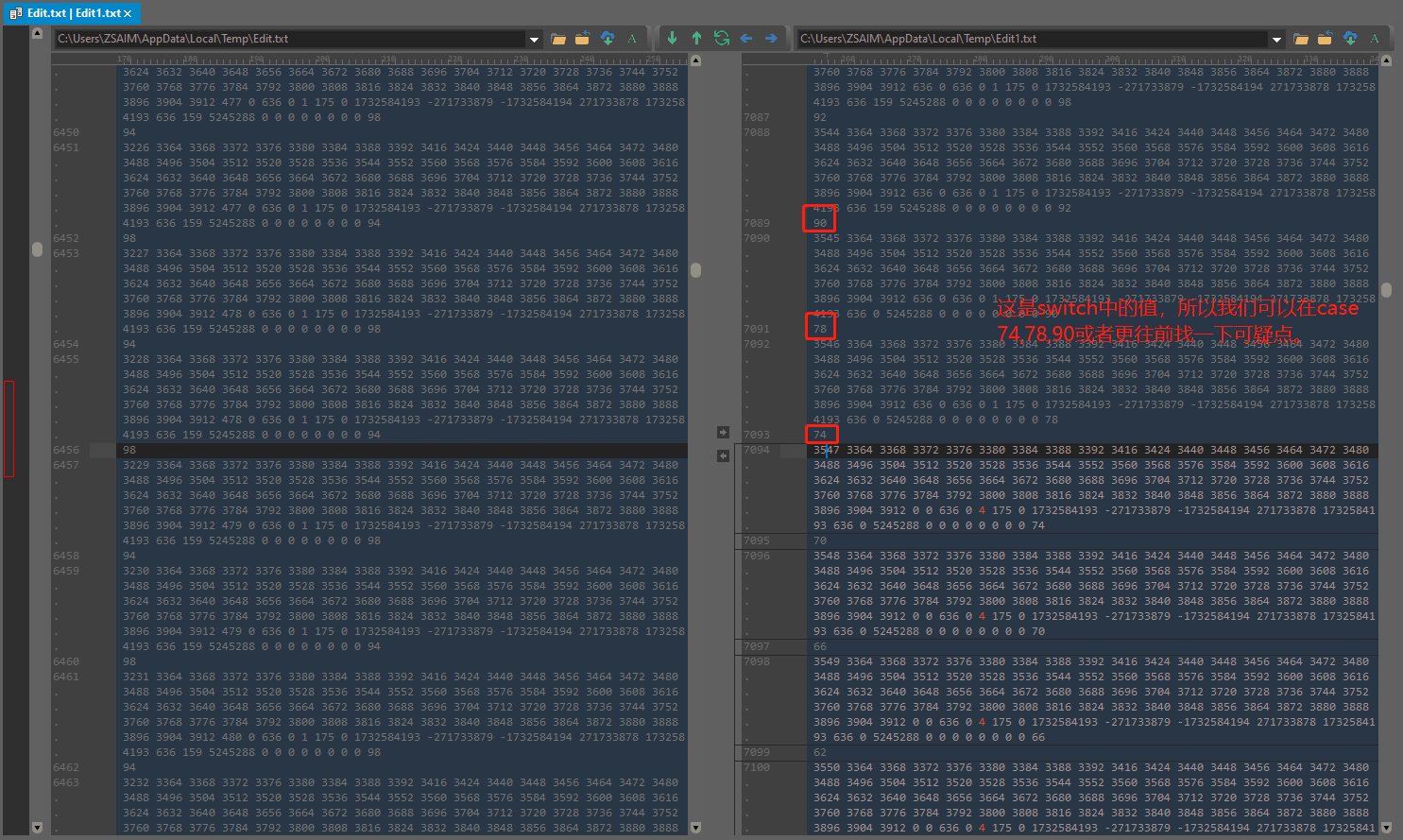•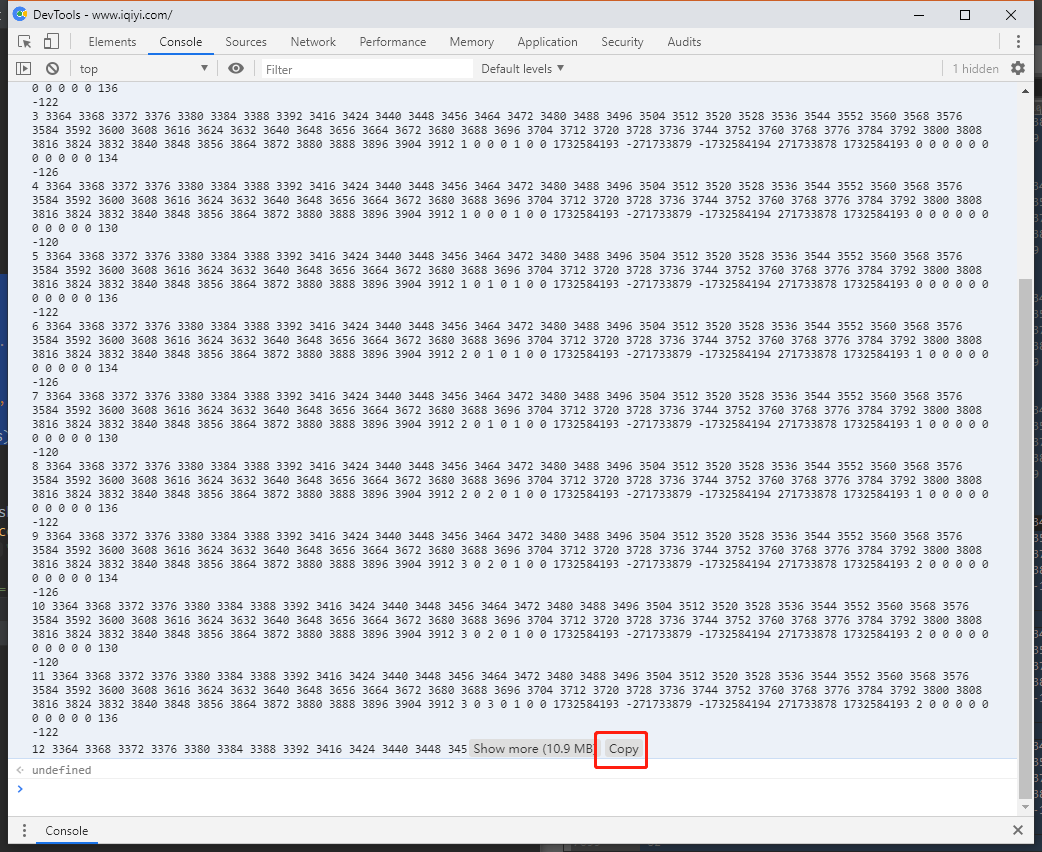•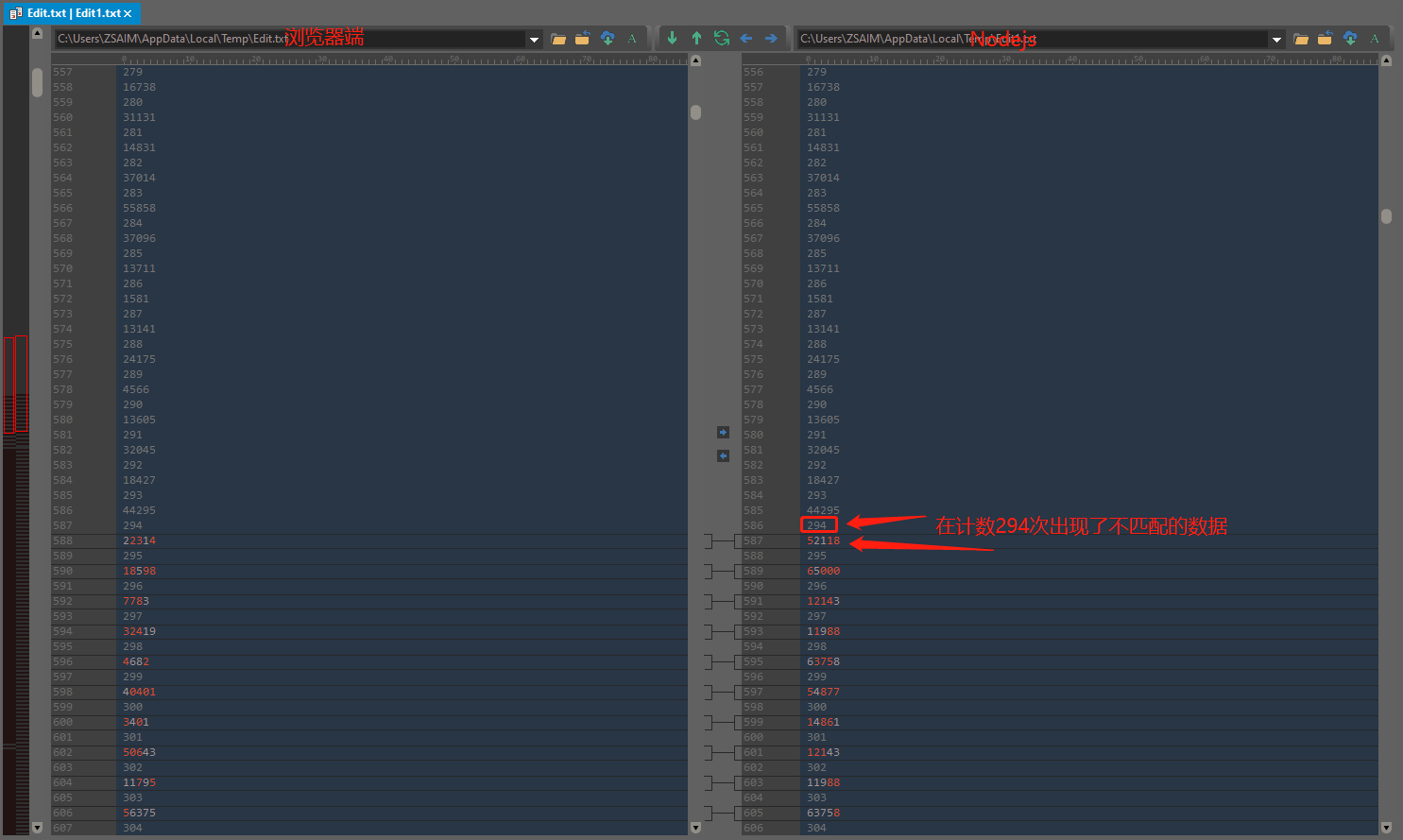• 通过一顿调试操作后，我们进入函数`function eV(){}`

• ``````function eV() {
var counter = 0;
for (var e = 27218; ; ) {
counter ++;
// 存储执行序列进行数据对比，以便找到“判断点”。
print_data.push([conter, e].join(' '));
switch (e) {
case 52924:

...
}
}
}
``````
• 然后再经过对比执行序列。

• 我们能找到如下用于检测nodejs的process。

• ``````case 59488:
try {
A = Object['prototype']['toString']['call'](C('process;')) === '[object process]'
} catch (e) {
}
e += -13840;
break;
``````
• ``````aD = function(aE) {
return eval(aE)
}
``````
• 可以看到的这里面会尝试运行代码`Object['prototype']['toString']['call'](C('process;'))`，也就是执行函数`aD()`, 然后执行返回`eval('process')`

• 这一条函数如果是在浏览器端将会产生异常，然后就会被`try catch()`捕捉，然后就会给`A`赋值，之后就会影响到接下来的算法结果。
• 如果是在非浏览器端nodejs执行这条函数对象`process`，也就是说他不会产生异常。
• 上面所说的就是一些所谓的非浏览器端的识别`开关`

• 如果有`process`对象，那么就会有不一样的`e`，之后就会执行不一样的流程。

#### 怎么处理呢？

• 解决方法其实很简单，只要将变量名改为一个在浏览器端和非浏览器端均产生异常或者均不产生异常的变量即可。这里可以直接将`process`改成`process1``require`改成`require1`就行了。

• 之后我们又找到了检测`require``document.domain`, `window.screen.clientWidth`, `window.screen.clientHeight`。。。。

• ``````case 40401:
A ? e += -37e3 : 'CESxF' === 'PPJkW' ? t = C('require;') : e += 11717;
break;
``````
• ``````case 6409:
try {
'NYIqF' === 'coDEu' ? (ba = g,
ca = s) : t = C('require;')
} catch (e) {
}
e += 52661;
break;
``````
• ``````case 37096:
A = C['clientHeight'],
e += -23385;
break;
``````
• 。。。
• 后面都是使用同样的方法，通过不断的对比数据序列来暴力调试。不断的修正，这就能得到最后的结果了。

• 经过了不断的调试，不断的对比序列，并且不断的修改，就能得到完全吻合的序列。

• 这种流程其实本质上这就是和软件的反汇编破解注册是一样的，只要在关键节点处进行修改跳转就行了。

### 随便说说

• `process``require`这些的检测，我们知道它都是可以经过异常捕获来进行浏览器端和非浏览器端的判断，所以这就可以让我们通过搜索被`try` `catch`的代码来进行分析是否存在这样的识别问题。
• 事实上，我们可以不经过反混淆就可以进行后面的所有操作，但是去掉混淆后会减轻很多调试负担。
• 正如前面所说的，我们可以进一步调试去掉库`jsdom`的依赖。但是这个并不是必须的。